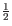## Calculation of the likelihood

After the prior and error probabilities have been estimated, the calculation of the likelihood is undertaken. For every combination of reference allele (site types) and nucleotide in every read, the probability of the observed allele being the same as the reference is calculated. These probabilities are then multiplied for all nucleotides in the reads at that position.

Here is an example:

Assumed reference allele: A/C

Read 1: C [(P(C|A)) +(P(C|C))] *

Read 2: C [( P(C|A)) +(P(C|C))] *

Read 3: A [( P(A|A)) +(P(A|C))] *

Read 4: A [( P(A|A)) +(P(A|C))] *

Read 5: T [( P(T|A)) +(P(T|C))]

Here, P(X|Y) is the probability that we will observe nucleotide X in a read when the true reference sequence is Y.